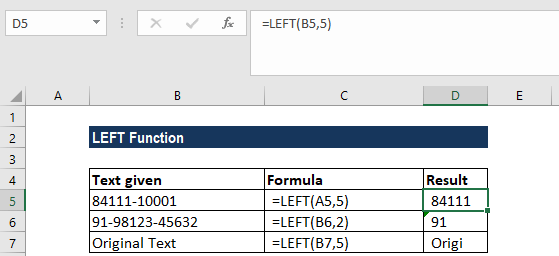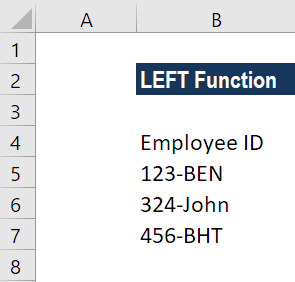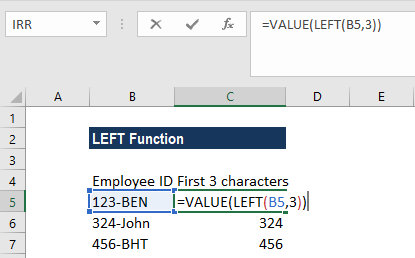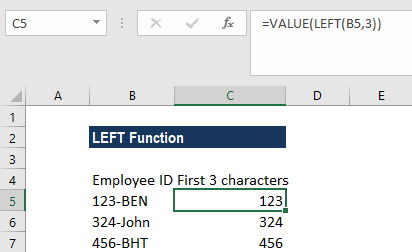# LEFT Function

Returns a specified number of characters from the start of a given text string

## What is the LEFT Function?

The LEFT Function is categorized under Excel TEXT functions. The function will return a specified number of characters from the start of a given text string.

In financial analysis, the LEFT function can be useful if we wish to extract characters from the left side of a text. Generally, it is used by combining it with other functions such as VALUE, SUM, COUNT, DATE, DAY, etc.

### Formula

=LEFT(text,[num_chars])

The LEFT function uses the following arguments:

1. Text (required argument) – This is the original text string.
2. Num_chars (optional argument) – Specifies the number of characters to be returned from the start of the given text. It is the number of characters to be extracted, starting from the left side of the text. If we omit the argument, the default value of 1 is taken.

### How to use the LEFT Function in Excel?

As a worksheet function, LEFT can be entered as part of a formula in a cell of a worksheet. To understand the uses of the function, let us consider an example:

#### Example 1

Suppose we wish to extract certain characters from the data below:

• 84111-10001
• 91-98123-45632
• Original Text

The results in Excel are shown in the screenshot below:#### Example 2

The LEFT function always returns a text string, even though it contains digits and looks like a number. This is important if we wish to use the result of the function within other functions or formulas.

Using the VALUE function, we can ensure that the number format is kept and the result is not converted to text.

Let us assume we wish to separate the numbers from the data below:We wish to extract the first 3 characters, that is, the numbers. The formula to use will be =VALUE(LEFT(B5,3)) as shown below:We get the results below:As we can see in the screenshot above, the numbers in column B obtained with a VALUE LEFT formula are right-alighted in the cells, as opposed to left-aligned text in column A. Since Excel recognizes the output as numbers, we can perform SUM or AVERAGE operations on the values, find the min and max value, or do other calculations.

### A few things to remember about the LEFT Function:

1. #VALUE! error – Occurs if the given [num_chars] argument is less than 0.
2. Dates are stored in Excel as numbers, and it is only the cell formatting that makes them appear as dates in our spreadsheet. Hence, if we attempt to use the LEFT function on a date, it will return the start characters of the number that represents that date.

For example, 01/01/1980 is represented by the number 29221, so applying the LEFT function to a cell containing the date 01/01/1980 (and requesting that 3 characters be returned) will result in a returned value of “292.” If we wish to use LEFT on dates, we can combine it with the DAY, DATE, MONTH, or YEAR functions. Otherwise, we can convert the cells containing dates to text using Excel’s Text to Columns tool.

Thanks for reading CFI’s guide to important Excel functions! By taking the time to learn and master these functions, you’ll significantly speed up your financial analysis. To learn more, check out these additional CFI resources:

• Excel Functions for Finance